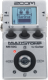# Deep chorus

Discussion in 'Zoom MS-50G' started by m-shim, May 15, 2022.

1. Deep chorusDevice: Zoom Ms-50g
Firmware: 3.10

Name on device: DeepChorus
Optimized for: Phones/Speaker

Effects chain:Chorus sound with deep plate reverb.

Effect: "ZNR" (Dynamics / Filter), active - "yes"
"THRSH" = 10
"DETCT" = GtrIn
"Level" = 100

Effect: "FD COMBO" (Amp simulator), active - "yes"
"Gain" = 20
"Tube" = 60
"Level" = 90
"Trebl" = 80
"Middl" = 70
"Bass" = 60
"Prese" = 60
"CAB" = ​
"OUT" = LINE

Effect: "ParaEQ" (Dynamics / Filter), active - "yes"
"Freq1" = 400Hz
"Q1" = 0.5
"Gain1" = -10
"Freq2" = 6.3kHz
"Q2" = 2
"Gain2" = 4
"Level" = 110

Effect: "SilkyCho" (Modulation / Sfx), active - "yes"
"LoMix" = 65
"HiMix" = 100
"ChMix" = 70
"LoPit" = 6
"HiPit" = 8
"PreD" = 27
"Rate" = 50
"Depth" = 46
"Tone" = 50

Effect: "Delay" (Delay / Reverb), active - "yes"
"Time" = 400
"F.B" = 15
"Mix" = 30
"HiDMP" = 5
"P-P" = P-P
"Level" = 100
"Tail" = On

Effect: "Plate" (Delay / Reverb), active - "yes"
"PreD" = 65
"Decay" = 50
"Mix" = 50
"Color" = 60
"LoDMP" = 50
"HiDMP" = 52
"Tail" = Off
"Level" = 100

Note: This is a patch file, you will need to download and install the ToneLib-Zoom software to use the patch.

File size:
464 bytes
Views:
109